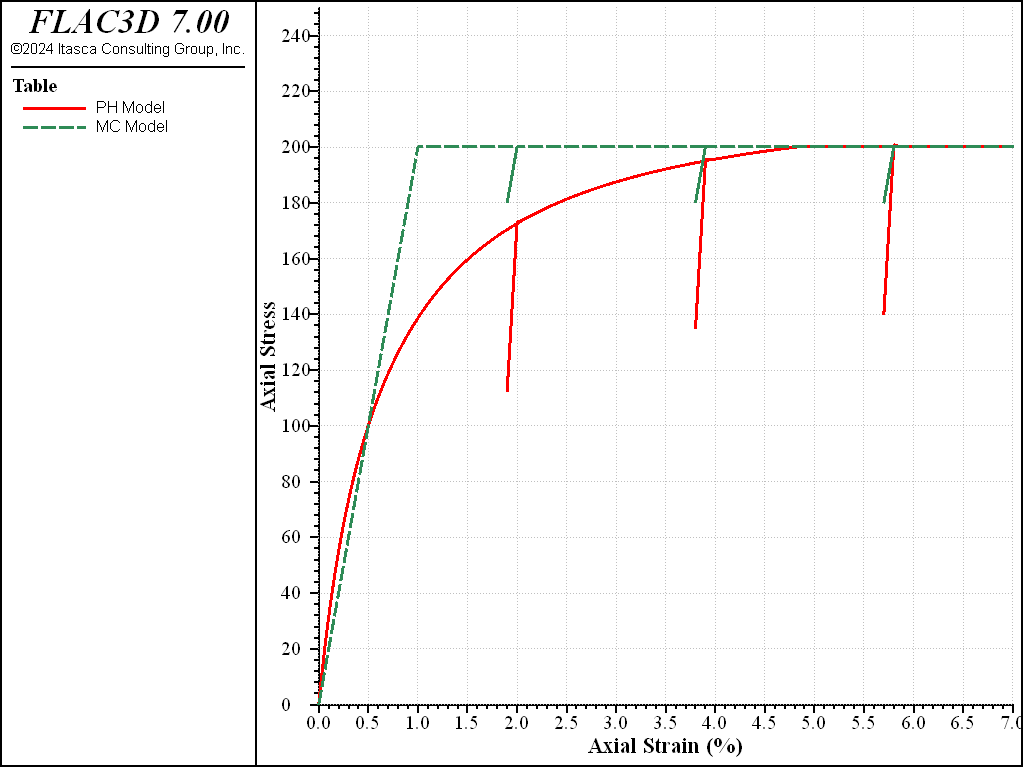FLAC3D Theory and Background • Constitutive Models

# Comparison between Mohr-Coulomb Model and Plastic-Hardening model

Note

To view this project in FLAC3D, use the menu command Help ► Examples…. Choose “ConstitutiveModels/ ComparisonPlasticHardening” and select “ComparisonPlasticHardening.prj” to load. The project’s main data files are shown at the end of this example.

This example compares the behavior of the Plastic-Hardening (PH) model and Mohr-Coulomb (MC) model during the triaxial compression. Both models are used in a one-zone triaxial compression test with a constant cell pressure of 100 kPa. The strength parameters (including friction angle, dilation angle, and tension limit) are the same for both models. The $$E_{50}$$ stiffness of the PH model is used as Young’s modulus for the MC model and $$E^{ref}_{ur}$$ is assumed to be three times the value of $$E^{ref}_{50}$$. Material properties for this example are summarized in Table 1. Material parameters not listed in the table are default values.

 Parameters PH MC $$\phi$$ (degrees) 30 30 $$c$$ (kPa) 0 0 $$\psi$$ (degrees) 10 10 $$E^{ref}_{50}$$ (kPa) 2e4 $$E$$ (kPa) 2e4 $$\nu$$ 0.2 0.2 $$E^{ref}_{ur}$$ (kPa) 6e4 $$m$$ 0.6 $$R_f$$ 0.9 $$p^{ref}$$ (kPa) 100 $$\sigma^{ini}_{1 \sim 3}$$ (kPa) -100

Figure 1 shows a plot deviatoric stress versus axial strain for both PH and MC models. It is easy to verify the following from the figure:

1. The ultimate failure deviatoric stresses (200 kPa) are the same for both models, as expected.
2. For the pre-failure curve, the PH and MC models are crossing at the half of the failure stress (100 kPa), which is consistent with the concept of $$E_{50}$$ stiffness.
3. The unloading stiffness in the MC model is the same as the loading stiffness ($$E$$ in the MC model, or $$E_{50}$$ in the PH model), while these stiffnesses are different in the PH model.Figure 1: Comparison of PH and MC models for a triaxial compression test.

Data Files

TriaxialCompressionPlasticHardening.dat

model new
model large-strain off
;
zone create brick size 1 1 1
zone cmodel assign plastic-hardening
zone property stiffness-50-reference=2.0e4 stiffness-ur-reference=6.0e4 ...
pressure-reference=100.0 exponent=0.6
zone property friction=30.0 dilation=10.0 cohesion=0.0
zone property stress-1-effective=-100.0 stress-2-effective=-100.0 ...
stress-3-effective=-100.0
;
zone gridpoint fix velocity-z
zone face apply stress-xx=-100.0 range union position-x 0 position-x 1
zone face apply stress-yy=-100.0 range union position-y 0 position-y 1
zone initialize stress xx -100.0 yy -100.0 zz -100.0
;
fish define hhhq_
local gp_ = gp.find(8)
global hhhq_ = zone.stress.xx(zp_) - zone.stress.zz(zp_)
global hhha_ = -gp.disp.z(gp_)
end
history interval 10
fish history hhhq_
fish history hhha_
;
zone gridpoint initialize velocity-z -2e-6 range position-z 1
model step 10000
zone gridpoint initialize velocity-z  1e-6 range position-z 1
model step  1000
zone gridpoint initialize velocity-z -2e-6 range position-z 1
model step 10000
zone gridpoint initialize velocity-z  1e-6 range position-z 1
model step  1000
zone gridpoint initialize velocity-z -2e-6 range position-z 1
model step 10000
zone gridpoint initialize velocity-z  1e-6 range position-z 1
model step  1000
zone gridpoint initialize velocity-z -2e-6 range position-z 1
model step 20000
;
hist export  '1' vs '2' table 'ph_qs_100'
table 'ph_qs_100' export 'ph_qs_100' truncate
;
model save 'ph100'


TriaxialCompressionMohrCoulomb.dat

model new
model large-strain off
;
zone create brick size 1 1 1
zone cmodel assign mohr-coulomb
zone property young=2.0e4 poisson=0.2 friction=30 dilation=10
;
zone gridpoint fix velocity-z
zone face apply stress-xx=-100.0 range union position-x 0 position-x 1
zone face apply stress-yy=-100.0 range union position-y 0 position-y 1
zone initialize stress xx -100.0 yy -100.0 zz -100.0
;
fish define hhhq_
local gp_ = gp.find(8)
global hhhq_ = zone.stress.xx(zp_) - zone.stress.zz(zp_)
global hhha_ = -gp.disp.z(gp_)
end
history interval 10
fish history hhhq_
fish history hhha_
;
zone gridpoint initialize velocity-z -2e-6 range position-z 1
model step 10000
zone gridpoint initialize velocity-z  1e-6 range position-z 1
model step  1000
zone gridpoint initialize velocity-z -2e-6 range position-z 1
model step 10000
zone gridpoint initialize velocity-z  1e-6 range position-z 1
model step  1000
zone gridpoint initialize velocity-z -2e-6 range position-z 1
model step 10000
zone gridpoint initialize velocity-z  1e-6 range position-z 1
model step  1000
zone gridpoint initialize velocity-z -2e-6 range position-z 1
model step 20000
;
hist export  '1' vs '2' table 'mc_qs_100'
table 'mc_qs_100' export 'mc_qs_100' truncate
;
model save 'mc100'